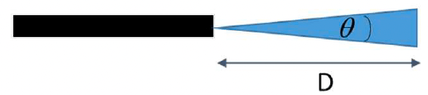# Intensity of a laser beam at a certain distance

• lorenz0
In summary, the angular divergence of a laser is the angle between the central beam and the radiation emanating from the perimeter of the laser beam, measured in radians.

#### lorenz0

Homework Statement
A laser emits radiation of wavelength ##\lambda= 632.8 nm## and a power of ##3 mW## and its beam has an angular divergence equal to ##180 \mu rad##. What is the intensity of the laser beam at a distance of ##D = 5 m##?
What would be the power of an isotropic source that provided the same intensity at the same distance?
Relevant Equations
##\theta=\frac{2R}{D}##, ##I=\frac{P}{4\pi d^2}##
I haven't been able to pinpoint a definition of "angular divergence" of a laser so by pure intuition I assumed it would be something like ##\theta=\frac{2R}{D}## and with that I have that the radius of the lase beam is ##R=\frac{D\theta}{2}=90\cdot 5 \cdot 10^{-6} m=450\ \mu m## so ##I_{5m}=\frac{P}{4\pi R^2}=\frac{3\cdot 10^{-3}}{4\pi \cdot 450^2 \cdot 10^{-12}}\simeq 1179\ \frac{Watt}{m^{2}}## and so I get for an isotropic source ##P_{iso}=I_{5m}\cdot 4\pi D^2=1179\cdot 4\pi 5^2 Watt=370391 Watt##. Now, I am suspicious of these results since I never had to use the wavelength \lambda so I think my definition of angular divergence is wrong; could anyone shed some light on this definition and how to apply it in this case? Thank you very much.

#### Attachments

•laser.png
4 KB · Views: 62
Last edited:
Hi,

And checking your math is always a good idea $$R=\frac{D\theta}{2}=90\cdot 5 \cdot 10^{-6} \ {\sf m}=450\ {\sf nm}\quad ?$$

##\ ##

Checking dimensions is always a good idea too $$I=\frac{I}{4\pi D^2}=\frac{3\cdot 10^{-3}}{4\pi 5^2}\ {\sf W}\simeq 9.6\cdot 10^{-6}\ {\sf W}\qquad ?$$

More tips: don't use ##I## and ##I## if ##I\ne I## !

##\ ##

•DaveE
BvU said:
Hi,

And checking your math is always a good idea $$R=\frac{D\theta}{2}=90\cdot 5 \cdot 10^{-6} \ {\sf m}=450\ {\sf nm}\quad ?$$

##\ ##
What I meant by "I haven't been able to pinpoint a definition" is that I found several definitions and since I didn't know the correct one to use in this case I went with the one that intuitively made the most sense.
------
Still, I don't understand why the problem gives ##\lambda## if it is of no use in the solution.

lorenz0 said:
so I get for an isotropic source ##I=\frac{P}{4\pi D^2}=\frac{3\cdot 10^{-3}}{4\pi 5^2}\ \frac{Watt}{m^2}\simeq 9.6\cdot 10^{-6}\ \frac{Watt}{m^2}##.
I don't understand this calculation. You seem to have found the intensity (##Wm^{-2}##, not ##W##) that would have been obtained with the same power source had it been isotropic. That is not what you are asked to find.

lorenz0 said:
I found several definitions
In such a case, (i.e. if your notes or textbook don't provide one) you state the definition and work with that, as you did. If I were to grade it (and notes or textbook indeed don't provide one), I wouldn't subtract points.

And the first hit (gentec) agrees with your intution, so my 'almost' was in error !

Now on to $$I_{5m}=\frac{P}{4\pi R^2}$$This is for an isotropic source. But not for your laser!

haruspex said:
I don't understand this calculation. You seem to have found the intensity (##Wm^{-2}##, not ##W##) that would have been obtained with the same power source had it been isotropic. That is not what you are asked to find.
Thanks, it should be correct now.

BvU said:
Now on to $$I_{5m}=\frac{P}{4\pi R^2}$$This is for an isotropic source. But not for your laser!
That R is not the distance from the source. D is used for that here.

BvU said:
Now on to $$I_{5m}=\frac{P}{4\pi R^2}$$This is for an isotropic source. But not for your laser!
I don't know the formula for the non-isotropic case so I thought I could modify that one and use as radius the one I got from the angular divergence.

lorenz0 said:
I am suspicious of these results since I never had to use the wavelength \lambda so I think my definition of angular divergence is wrong;
The item on Gaussian laser beams at https://en.m.wikipedia.org/wiki/Beam_divergence shows how the wavelength can be relevant, but since you are not given the aperture at source it does not seem relevant here.

haruspex said:
The item on Gaussian laser beams at https://en.m.wikipedia.org/wiki/Beam_divergence shows how the wavelength can be relevant, but since you are not given the aperture at source it does not seem relevant here.
That is exactly what I thought too.

lorenz0 said:
Thanks, it should be correct now.
Doesn’t seem quite right. It has to illuminate an area ##4\pi D^2## instead of ##\frac 14\pi(D\theta)^2##. So I get ##3mW*\frac{16}{\theta^2}##, which is about 1.5MW.

@lorenz0 : you grasp this factor 4 now ?

Final note: when you get input of 1 or 2 digit accuracy, don't provide a result wih 6 digits.

##\ ##